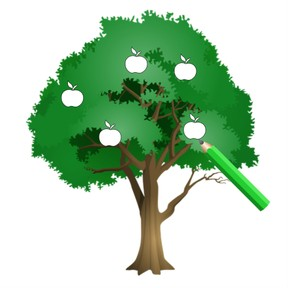Draw quantities to 5

# Draw quantities to 5

Draw quantities to 5

No account needed.8,000 schools use Gynzy92,000 teachers use Gynzy1,600,000 students use Gynzy

## General

Students can draw quantities from 1-5. They know which number belongs to which quantity.

K.CC.B.5

## Relevance

Discuss with students that it is important to be able to draw quantities to 5 so that they know which number belongs to each quantity. If someone turns four, and you make them a drawing with five candles, that isn't right. It is more fun to get a drawing with the right number of candles on it.

## Introduction

Ask a few students, maximum of 5, to come to the front of the class. Ask the students how many are standing in front. Then set a maximum of 5 chairs in the front of the class and ask the students how many chairs there are.

## Development

The interactive whiteboard has an image of an aquarium with a fish in it. Point at the fish and say the number 1. Under the fish is the number 1. The next image shows an aquarium. Point at the fish, and count the number aloud. Repeat this until there are five fish in the aquarium. To check that students understand how to draw quantities, show them a tree and say that the tree needs to have five apples drawn in it. Ask if they know how they can do this. Draw the apples. Do the same with the tree that needs one apple drawn. Ask students to draw ice cream cones with the right number of scoops. They can hold up their drawings to check their answers. Next students have to indicate how many balloons are drawn. Ask the students to count out loud.

Check that students can draw the correct number of objects by asking the following questions:
How do you know how many objects have been drawn?
How can you draw the right number of objects?

## Guided Practice

Students use a worksheet to practice drawing quantities to 5

## Closing

Repeat that it is useful for students to know how to draw quantities to 5 so that they know which number belongs to each quantity. By doing so they can draw the right number of candles on a cake for a friend's birthday. Ask the students to practice drawing quantities in pairs. First, one student writes a number from one to 5. Their partner must draw the right number of objects. They take turns writing numbers and drawing.

## Teaching Tip

Students who have difficulty drawing quantities can write the numbers 1 to 5 under the objects that they have drawn. That way they see the number line and know which number belongs to which quantity.

### The online teaching platform for interactive whiteboards and displays in schools

• Save time building lessons

• Manage the classroom more efficiently

• Increase student engagement